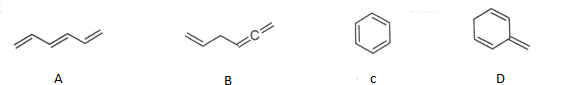Problem: From the compounds listed below, which has the lowest heat of hydrogenation. a.B b.E c.D d.A e.C   F. All of the above are the same.

FREE Expert Solution
84% (469 ratings)
Problem Details

From the compounds listed below, which has the lowest heat of hydrogenation.

a.B
b.E
c.D
d.A
e.C

F. All of the above are the same.What scientific concept do you need to know in order to solve this problem?

Our tutors have indicated that to solve this problem you will need to apply the Alkene Stability concept. You can view video lessons to learn Alkene Stability Or if you need more Alkene Stability practice, you can also practice Alkene Stability practice problems .

What is the difficulty of this problem?

Our tutors rated the difficulty of From the compounds listed below, which has the lowest heat o... as high difficulty.

How long does this problem take to solve?

Our expert Organic tutor, Chris took 3 minutes to solve this problem. You can follow their steps in the video explanation above.

What professor is this problem relevant for?

Based on our data, we think this problem is relevant for Professor Schmeltzer's class at CCNY.